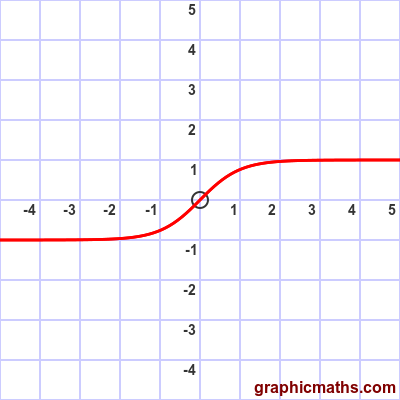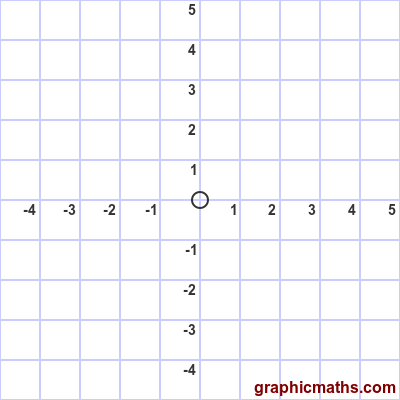# tanh function

By Martin McBride, 2020-09-10
Tags: tanh hyperbolic function
Categories: hyperbolic functions pure mathematicsThe tanh function is a hyperbolic function. It is also known as the hyperbolic tangent function.

Here is a video that explains sinh, cosh and tanh:

## Equation and graph

The tanh function is defined as sinh divided by cosh:

$$\tanh{x} = \frac{\sinh{x}}{\cosh{x}}$$

Here is a graph of the function:An alternative equation is:

$$\tanh{x} = \frac{e^{x}-e^{-x}}{2} \times \frac{2}{e^{x}+e^{-x}} = \frac{e^{x}-e^{-x}}{e^{x}+e^{-x}}$$

## tanh as sinh divided by cosh

The tanh function involves two functions, $\sinh{x}$ divided by $\cosh{x}$. This animation illustrates this:When $x$ is zero, $\sinh x$ is 0 and $\cosh x$ is 1, so the result is 0.

As $x$ gets larger, as the animation shows, $\sinh x$ and $\cosh x$ become ever closer is value, although $\sinh x$ is always slightly smaller. The value of $\tanh x$ therefore tends towards 1.

When $x$ is large and negative, $\sinh x$ becomes ever closer to $-\cosh x$. The value of $\tanh x$ therefore tends towards -1.

## Other forms of the equation

If we take the equation:

$$\tanh{x} = \frac{e^{x}-e^{-x}}{e^{x}+e^{-x}}$$

and multiply top and bottom by by $e^{x}$ we get:

$$\tanh{x} = \frac{e^{2x}-1}{e^{2x}+1}$$

See the similar derivation for the sinh function

An alternative form is obtained by multiplying top and bottom by by $e^{-x}$:

$$\tanh{x} = \frac{1-e^{-2x}}{1+e^{-2x}}$$